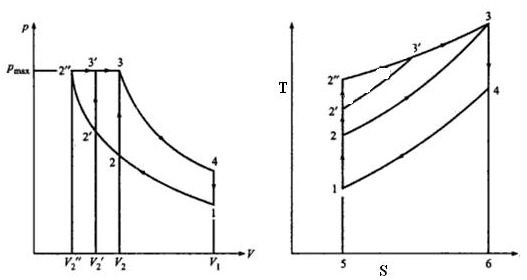# Diesel engine cycle comparison – otto cycle vs other cycles for marine diesel engines

## Introduction

In these three articles we studied about Otto cycle, diesel cycle and dual cycle and looked at their thermal efficiency. In this article we will take a collective look at these three cycles in order to compare and contrast them, so that we can come to know the relative advantages and disadvantages of these cycles.

## The 3 Cycles Compared

In the last article we compared the three cycles their compression ratio and heat rejection were kept constant for all cycles. In this article we will focus on peak pressure, peak temperature and heat rejection. The P-V and T-S diagrams of these three cycles for such a situation are drawn simultaneously as described below.In the above diagrams the following are the cycles

• Otto cycle: 1 – 2 – 3 – 4 – 1
• Dual cycle: 1 – 2’ – 3’ – 3 – 4 – 1
• Diesel cycle: 1 – 2” – 3 – 4 – 1

Remember that we are assuming the same peak pressure denoted by Pmax on the P-V diagram. And from the T-S diagram we know that T3 is the highest of the peak temperature which is again same for all three cycles under consideration. Heat rejection given by the area under 4 – 1 – 5 – 6 in the T-S diagram is also same for each case.

In this case the compression ratio is different for each cycle and can be found by dividing V1 with the respective V2 volumes of each cycle from the P-V diagram. The heat supplied or added in each cycle is given by the areas as follows from the T-S diagram

• Otto cycle: Area under 2 – 3 – 6 – 5 say q1
• Dual cycle: Area under 2’ – 2’ – 3 – 6 – 5 say q2
• Diesel cycle: Area under 2” – 3 – 6 – 5 say q3

It can also be seen from the same diagram that q3>q2>q1

We know that thermal efficiency is given by 1 – heat rejected/heat supplied

Since heat rejected is same and we know the order of magnitude of heat supplied, we can combine this information to conclude that

Thermal efficiency of these engines under given circumstances is of the following order

Diesel>Dual>Otto

Hence in this case it is the diesel cycle which shows greater thermal efficiency.